Laminar Flow

# Laminar Flow Notes | Study Civil Engineering SSC JE (Technical) - Civil Engineering (CE)

## Document Description: Laminar Flow for Civil Engineering (CE) 2022 is part of Civil Engineering SSC JE (Technical) preparation. The notes and questions for Laminar Flow have been prepared according to the Civil Engineering (CE) exam syllabus. Information about Laminar Flow covers topics like and Laminar Flow Example, for Civil Engineering (CE) 2022 Exam. Find important definitions, questions, notes, meanings, examples, exercises and tests below for Laminar Flow.

Introduction of Laminar Flow in English is available as part of our Civil Engineering SSC JE (Technical) for Civil Engineering (CE) & Laminar Flow in Hindi for Civil Engineering SSC JE (Technical) course. Download more important topics related with notes, lectures and mock test series for Civil Engineering (CE) Exam by signing up for free. Civil Engineering (CE): Laminar Flow Notes | Study Civil Engineering SSC JE (Technical) - Civil Engineering (CE)
 1 Crore+ students have signed up on EduRev. Have you?

Chapter 10 Laminar Flow

• Fluid particles move along straight parallel paths in layers or laminae
•  It occurs at low velocity; Viscosity force predominates inertial force.
•  Relation between Shear and Pressure Gradients in Laminar Flow
•  For a steady uniform flow,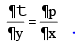• Thus, for a steady uniform laminar flow the pressure gradient in the direction of flow is equal to the shear stress gradient in the normal direction.
• By using newton’s law of viscosity :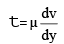• The differential equation of laminar flow is given by,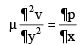• Steady Laminar Flow in Circular Pipes (Nagen - Poiseulle flow)
• In a circular pipe with steady laminar flow, the shear stress t varies linearly along the radius of the pipe as,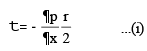• The maximum value of strees t0 occurs at r = R (i.e., at the centre of pipe),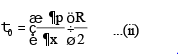• The negative sign on (p/x) indicates decrease in pressure in the direction of flow. The pressure must decrease  because pressure force is the only means available to compensate for resistance to the flow, the potential  and kinetic energy remain constant.
• In laminar flow shear stress is entirely due to viscous action. Hence by Newton’s law of viscosity.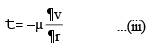• By combining (i) and (iii) and integrating under proper limits, we get,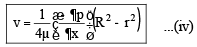which shows that for laminar flow through circular pipe, velocity of flow varies parabolically and the
surface of velocity distribution is a paraboloid of revolution.

• The maximum velocity occurs at axis and is given by,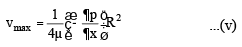• By combining equal (iv) and (v), the velocity distribution in laminar flow is obtained as,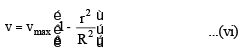• The corresponding expression for discharge is given by,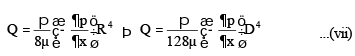This equation is called Hagen Poiseulle equation.

• The mean velocity of flow (V) is given by,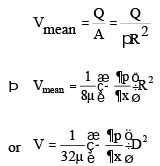.                  ..............(viii)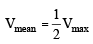........ ...(ix)

• The point where local velocity is equal to mean velocity is given by,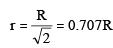so mean velocity of flow occurs at a radial distance of 0.707 R from the centre of the pipe.

• The velocity and shear stress distribution are as shown below :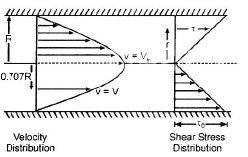Laminar Flow Between Parallel Plates
Case 1 : Both plates are at Rest

• For the laminar flow of fluid between two fixed parallel flat plates located at distance B apart, the velocity

distribution is given by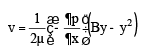...(i)

• The maximum velocity occurs at y = (B/2) and it is given by,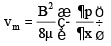...(ii)

• The discharge q per unit width of plate is given by,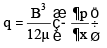...(iii)

• The mean velocity of flow is given by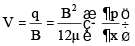........(iv)

By comparing (ii) and (iv)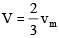....(v)

• The pressure drop between any two points distance L apart is given by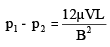...(vi)

• The distribution of shear stress is given by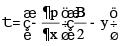...(vii)

• The shear stress is maximum at y = 0 and is given by :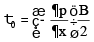...(viii)

• The velocity and shear stress distribution is as shown below :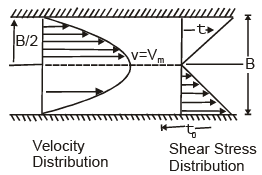Case 2 : When one Plate Moving And Other at Rest is known as COUETTE Flow

• The velocity and stress distribution in COUETTE FLOW is shown below :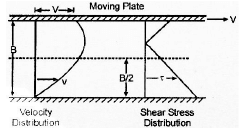Expression For Head Loss
(a) In case of laminar flow through pipes :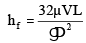(b) In case of laminar flow through parallel plates :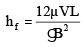(c) In case of open channel flow :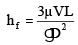(d) The general equation is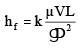where,
hf = loss of head in length L
V = mean velocity of flow
D = characteristic dimension representing the geometry of passage.
k = constant, whose value depends upon the shape of passage.

The document Laminar Flow Notes | Study Civil Engineering SSC JE (Technical) - Civil Engineering (CE) is a part of the Civil Engineering (CE) Course Civil Engineering SSC JE (Technical).
All you need of Civil Engineering (CE) at this link: Civil Engineering (CE)

## Civil Engineering SSC JE (Technical)

2 videos|111 docs|50 tests
 Use Code STAYHOME200 and get INR 200 additional OFF

## Civil Engineering SSC JE (Technical)

2 videos|111 docs|50 tests

Track your progress, build streaks, highlight & save important lessons and more!

,

,

,

,

,

,

,

,

,

,

,

,

,

,

,

,

,

,

,

,

,

;